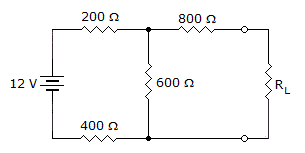# Electronics - Series-Parallel Circuits - Discussion

### Discussion :: Series-Parallel Circuits - True or False (Q.No.4)

4.In the given circuit, the Thevenin resistance equals 800.

 [A]. True [B]. False

Explanation:

No answer description available for this question.

 Radhika said: (Oct 20, 2013) When we short 12V we get (400+600) ohm resistance then 600 parallel with 600 gives 300 ohm finally we get 300+800 ohm i.e. 1100 ohm.

 Kiran V said: (Jul 26, 2020) ,When we short 12V we get (200+400) ohm resistance , then 600 parallel with 600 gives 300 ohm finally we get 300+800 ohm i.e. 1100 ohm.